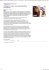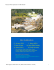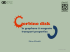# view as pdf

## Transcription

view as pdf
```Electronic properties of corrugated graphene.
F.Guinea
A. Castro-Neto (Boston U.), N. M. R. Peres (U. Minho, Portugal), E. V. Castro, J. dos Santos (Porto), J. Nilsson (Boston U.), A. Morpurgo
(Delft), M. I. Katsnelson (Nijmegen), D. Huertas-Hernando (Trondheim, Norway), D. P. Arovas, M. M. Fogler (U. C. San Diego), F.
von Oppen (Berlin), A. Akhmerov (Leyden), J. González, F. G., G. León, M. P. López-Sancho, T. Stauber, J. A. Vergés, M. A.
H. Vozmediano, B Wunsch (CSIC, Madrid), A. K. Geim, K. S. Novoselov (U. Manchester), A. Lanzara (U. C. Berkeley), M. Hentschel
(Dresden), E. Prada, P. San-José (Karlsruhe, Lancaster), J. L. Mañes (U. País Vasco, Spain), F. Sols (U. Complutense, Madrid), E. Louis
(U. Alicante, Spain), A. L. Vázquez de Parga, R. Miranda (U. Autónoma, Madrid), B. Horovitz (Beersheva), P. Le Doussal (ENS, Paris),
A. K. Savchenko (Exeter).
KITPGraphene week.
Apr. 13-17, 2009
Midgap states in graphene quantum dots (with A.
Akhmerov)
Effective electric fields (with F. von Oppen, E.
Mariani)
Effective and real magnetic fields in suspended
graphene samples (with M. Fogler, M. I. Katsnelson, G.
León, E. Prada, P. San José, A. K. Geim)
Localized states at edges
2
M. Fujita, K. Wakabayashi, K. Nakada, and K. Kusakabe,
Journ. Phys. Soc. Jap. 65, 1920 (1996)
N. M.. R. Peres, F. G., and A. H. Castro Neto, Phys. Rev. B
73, 125411 (2006)
Boundary conditions at edges
A. R. Akhmerov and C. W. J. Beenakker, Phys. Rev. B 77, 085423 (2008)
M. V. Berry and R. J. Mondragon, Proc. R. Soc. London Ser. A 412, 53 (1987),
E. McCann and V. I. Fal’ko, J. Phys.: Condens. Matter 16, 2371, (2004),
L. Brey and H. A. Fertig Phys. Rev. B 73, 235411 (2006)
From A. R. Akhmerov and C. W. J. Beenakker, Phys. Rev. B 77, 085423 (2008)
3
Experiments
J. Güttinger, C. Stampfer, S. Hellmüller, F. Molitor, T. Ihn
and K. Ensslin, Appl. Phys. Lett. 93, 212102 (2008),
C. Stampfer, J. Güttinger, F. Molitor, D. Graf, T. Ihn, and
K. Ensslin, Appl. Phys. Lett., 92, 012102 (2008).
C. Stampfer, E. Schurtenberger, F. Molitor, J. Gttinger, T.
Ihn, and K. Ensslin, Nano Lett. 8, 2378 (2008).
4
L. A. Ponomarenko, F. Schedin, M. I.
Katsnelson, R. Yang, E. H. Hill, K. S.
Novoselov, A. K.Geim, Science 320, 356
(2008)
Ballistic quantum dots
θ
Ψi (r|| , r⊥ ) ∝ e
L’
5
ik i r||
e −λi r⊥
vF −λi L′
e
L
2π
4π
1
, c(θ )
, ... ,
λi ≈ c(θ )
L′
L′
l (θ )
εi ≈
L
⎧ 1
⎪⎪ ε
D(ε ) = ∑ δ (ε − ε i ) ≈ ⎨ 2
εL
i
⎪ 2
⎪⎩ vF
D(ε )
N* ≈
ε
vF
L
L
l
vF − L l
v
e
≤ε ≤ F
L
L
vF
≤ε
L
Edges in graphene
6
A. L. Vázquez de Parga, F. Calleja, B. Borca, M. C.
G. Passeggi, Jr., J. J. Hinarejos, F. G., and R. Miranda,
Phys. Rev. Lett. 100, 056807 (2008)
X. Jia, M. Hofmann, V. Meunier, B. G. Sumpter, J. Campos-Delgado,
J. M. Romo-Herrera, H. Son, Y.-P. Hsieh, A. Reina, J. Kong, M.
Terrones, M. S. Dresselhaus, Science 323, 1701 (2009)
Ç. Ö. Girit, J. C. Meyer, R.Erni, M. D.
Rossell, C. Kisielowski, L. Yang, C.-H. Park,
M. F. Crommie, M. L. Cohen, S. G. Louie, A.
Zettl, Science 323, 1705 (2009)
7
Ballistic quantum dots. Edge potentials
C
O, H
⎧V0
V (r|| , r⊥ ) = ⎨
⎩0
r⊥ < d
r⊥ ≥ d
vF −λi L
ε i = e + V0 λi d + t ′a 2 λi2
L
W * = Max(V0 , t ′)
D(ε )
l * = Max(l , d )
ε
⎧ L 1 ε L2
⎪ * *+ 2
vF
⎪l W
D(ε ) ≈ ⎨
2
L
ε
⎪
⎪⎩
vF2
0 ≤ ε ≤W*
W* ≤ε
Ballistic quantum dots. Localized states
N
ε
vF2
l* W *
2
*
*
L
2
⎞
⎛
v
*
*
F
ε ≈ Min⎜⎜W , * * ⎟⎟
Ll W ⎠
⎝
2
⎞
⎛
L
v
*
F
N ≈ Min⎜ * , 2 2 ⎟
⎜ l l* W * ⎟
⎠
⎝
2
⎞
⎛L
v
L
*
F
⎟
N ribbon ≈ Min⎜ * , 2 2
⎜ l l * W * Wribbon ⎟
⎠
⎝
There are approximately
N* states localized at the
edges, within a range ε*
of the Dirac point
L ≈ 1 μm
l * ≈ 0.2 nm
W * ≈ 0.3 eV
N * ≈ 100
ε ≈ 6 meV
*
8
9
Effective gauge fields
rr
r r
r r
ik3 a3 ⎞
ik1a1
ik 2 a 2
⎛
0
+
+
t
e
t
e
t
e
1
2
3
⎟≈
r
r
H ≡ ⎜ −ikr ar
⎜ t e 1 1 + t e −ik 2 ar2 + t e −ik3ar3
⎟
0
2
3
⎝1
⎠
3t a
⎛
(k x + ik y ) + Δt ⎞⎟
0
⎜
2
⎟
⎜
3
t
a
⎟⎟
⎜⎜
(k x + ik y ) + Δt
0
⎠
⎝ 2
K'
K
K
K'
K'
K
K
K'
A modulation of the hoppings leads to
a term which modifies the momentum:
an effective gauge field.
The induced “magnetic” fields have
opposite sign at the two corners of the
Brillouin Zone.
K
K
K'
K'
These terms are forbidden by symmetry in
clean graphene.
Effective electric fields
10
⎛
V ∑ uii
vF (− i∂ x − ∂ y − Ax + iAy )⎞
⎜
⎟
i
HK ≡⎜
⎟
(
)
v
i
A
iA
V
u
−
∂
+
∂
−
−
∑
F
x
y
x
y
ii
⎜
⎟
i
⎝
⎠
Ax =
β
2a
(u
xx
− u yy )
Ay =
2u xy
∂ log(t )
≈ 2−3
∂ log(a )
H. Suzuura and T. Ando, Phys. Rev. B 65, 235412 (2002)
J. L. Mañes, Phys. Rev. B 76, 045430 (2007)
r
r
⎛
⎞ ∂A
E = −V∇⎜ ∑ uii ⎟ ±
⎝ i
⎠ ∂t
r
r
⎛ r2 q
Nβ r ⎞ r
±
Eqr ≈ ⎜⎜V q ×
q ωqr ⎟⎟ B r
q
k FT 2a
⎝
⎠
r
ωqr = c q ≈
D. García-Sánchez, A. M. van der Zande, A. San
Paulo, B. Lassagne, P. L. McEuen, A. Bachtold,
Nano Lett. 8, 1399 (2008)
2a
β=
V ≈ 10 − 20 eV
J. Scott Bunch, A. M. van der Zande, Scott S. Verbridge, I.
W. Frank, D. M. Tanenbaum, J. M. Parpia, H. G.
Craighead, P. L. McEuen, Science 315, 490 (2007)
β
E gauge
Escalar
c
L
screening
θ Debye
c a −1
≈ Nβ
≈ Nβ
k FT L >> 1
V
V
k FT L
Energy dissipation
τ τV
D=v
τ +τV
σ DC = ν D
11
2
F
1
τV
≈
1
τV 0
T2
+c
EF
dW r r
= j E = ωqr Γqr
dt
Valley drag:
K. Flensberg, B. Y.-K. Hu, A.-P. Jauho, J. M. Kinaret, Phys. Rev. B 52, 14761 (1995)
A. Kamenev, Y. Oreg, Phys. Rev. B 52, 7516 (1995)
W.-K. Tse, Y.-K. Yu, and S. Das Sarma, Phys. Rev. B.76, 081401 (2008)
r
EK
r
EK ′
r
EK ′
r
q
Longitudinal polarization
r
EK
r
q
Transverse polarization
Conductivity
12
See: B. I. Halperin, Patrick A. Lee, and N. Read, Phys. Rev. 47, 7312 (1993)
r
∂ nK
1
+ ∇ jK = − (nK − nK ′ )
∂t
τV
Continuity
r
∂ nK ′
1
+ ∇ jK ′ = − (nK ′ − nK )
∂t
τV
r
σ || (q , ω ) = σ DC
Ohm’s law
⎛1 1
⎜⎜ +
⎝ τ τV
1 r
−
jK
τV
⎞r
1 r
vF2
e 2ν vF2 r
⎟⎟ jK −
j K ′ = − ∇ nK +
EK
2
2
τ
V
⎠
⎛1 1 ⎞ r
vF2
e 2ν vF2 r
+ ⎜⎜ + ⎟⎟ jK ′ = − ∇ nK ′ +
EK ′
2
2
τ
τ
V ⎠
⎝
⎡ 2 2 ⎛ r 2 2 ⎞⎤
⎢ω + ⎜⎜ D q + ⎟⎟⎥
τV ⎝
τ V ⎠⎦
⎣
2
⎛
⎞
r
2
2
ω 2 + ⎜⎜ D q + ⎟⎟
τV ⎠
⎝
Longitudinal polarization
r
σ ⊥ (q , ω ) = σ DC
Transverse polarization
r2
r
D q ↔ vF q
Ballistic limit:
r −1
l↔q
13
Quality factor
n = 1012 cm −2
2
1
T
+
τ V−1 =
10τ E F
L = q −1 = 1μ m
Longitudinal polarization
Transverse polarization
Semiclassical analysis
Valid for multilayered systems
Suspended graphene. Graphene membranes
J. S. Bunch, S. S. Verbridge, J. S.
Alden, A. M. van der Zande, J. M.
Parpia, H. G. Craighead, and P. L.
McEuen, Nano Lett. 8, 2458 (2008)
14
K. I. Bolotin, K. J. Sikes, Z. Jiang, G.
Fudenberg, J. Hone, P. Kim, and H. L.
Stormer, Solid St. Commun. 146, 351
(2008)
T. J. Booth, P. Blake, R. R. Nair, D.
Jiang, E. W. Hill, U. Bangert, A.
Bleloch, M. Gass, K. S. Novoselov, M.
I. Katsnelson, and A. K. Geim, Nano
Lett. 8, 2442 (2008)
X. Du, I. Skachko, A. Barker, E. Y.
Andrei, Nature Nanotechnology 3, 491
(2008)
Suspended graphene. Graphene membranes
K. S. Kim, Y. Zhao, H. Jang, S. Y. Lee, J. M. Kim, K. S. Kim, J. H. Ahn, P.
Kim, J.-Y. Choi, B. H. Hong, Nature 457, 706 (2008)
C. Lee, X. Wei, J. W. Kysar, J. Hone,
Science 321, 385 (2008)
W. Bao, F. Miao, Z. Chen, H. Zhang, W. Jang, C. Dames,
C. N. Lau, arXiv:0903.0414
15
Ballistic transport in suspended graphene
16
M. M. Fogler, F. G., M. I. Katsnelson, Phys. Rev. Lett.
101, 226804 (2008)
The graphene layer is
deformed by the applied electric
field, slack, …
gauge potentials
AyH cm-1L
h0H ÅL
200
8ä105
150
100
4ä105
50
3ä1011
6ä1011
r, cm-2
Maximum height as function
of carrier density for different
values of the slack
3ä1011
6ä1011
r, cm-2
Vector potential inside the
suspended region as function
of carrier density for different
values of the slack
Ballistic transport in suspended graphene
17
GH e2êhL
200
150
100
50
-6ä1011
-3ä1011
3ä1011
6ä1011
r, cm-2
Transmission through a deformed graphene sheet as
function of density for different values of the slack
Hall currents in a magnetic field
Effects of a real magnetic field
G. León, E. Prada, P. San José, F. G., unpublished
Landau levels as function of the
momentum parallel to the boundary
Integer Quantum Hall steps in a
graphene ribbon where part of it is under
a constant stress
18
Effective magnetic fields in strained graphene
19
A. K. Geim, M. I. Katsnelson, F. G., unpublished
u r (r , θ ) = Ar 2 sin (3θ )
uθ (r , θ ) = Ar 2 cos(3θ )
σ rr (r ,θ ) = 4μAr sin (3θ )
σ θθ (r ,θ ) = −4 μAr sin (3θ )
σ rθ (r ,θ ) = 4 μAr cos(3θ )
u x ( x, y ) = 2 Axy
(
u y ( x, y ) = A x 2 − y 2
σ xx (x, y ) = 4 μAy
σ yy (x, y ) = −4 μAy
σ xy ( x, y ) = 4μAx
lB ≈
)
Shear deformation:
Constant effective
magnetic field
aR
8u β
R ≈ 1μm, a ≈ 0.1nm, β ≈ 3, u ≈ 0.1
l B ≈ 7 nm, B ≈ 10T
DHEL
0.20
Applied forces
0.15
Dependence on
boundary
conditions:
0.10
0.05
-0.2
Effective magnetic field
-0.1
0.0
0.1
0.2
EHeVL
Total density of states
Strain superlattices
20
eV
0.10
0.08
0.06
Height modulations
0.04
0.02
G
M
K
Electronic bands
Effective magnetic field
G
Wrinkles and mechanical instabilities in strained graphene
F. G., B. Horovitz, P. Le Doussal, arXiv:0811.4670, Solid St. Commun., in press
Wrinkling instability:
E.Cerda and L. Mahadevan, Phys. Rev. Lett. 90, 074302 (2003)
T. A. Witten, Rev. Mod. Phys. 79, 643 (2007)
E.Cerda and L. Mahadevan, Phys. Rev. Lett. 90, 074302 (2003)
Possible instabilities in graphene on a substrate
21
22
Localized states at edges can lead to
observable consequences in graphene quantum
dots
Synthetic electric fields are induced in
vibrating graphene. They give a simple physical
picture of dissipation mechanisms.
Synthetic magnetic fields interfere with real
magnetic fields in suspended samples, modifying
the properties in the Quantum Hall regime.
```

### Göttinger Forscher entwickeln flexiblen Laufroboter